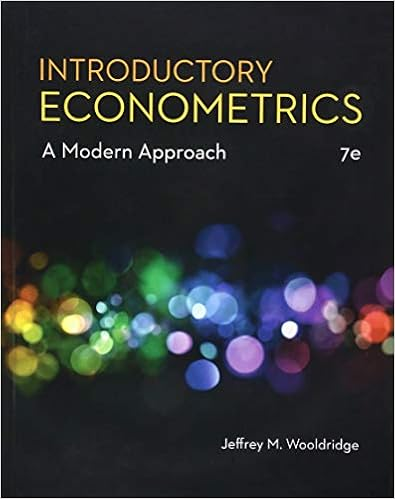# Stat 72 Test 3 Spring 2013 - STAT 72 TEST 3 NAME Use at...

• Test Prep
• 11

This preview shows page 1 - 3 out of 11 pages.

##### We have textbook solutions for you!
The document you are viewing contains questions related to this textbook.The document you are viewing contains questions related to this textbook.
Chapter 10 / Exercise C12
Introductory Econometrics: A Modern Approach
WooldridgeExpert Verified
STAT 72 – TEST 3NAME: _____________________________Use at least 4 decimal places. 1)The slope (b1) represents? (3 points)________predicted value of Y when X = 0____x___the estimated average change in Y per unit change in X________the predicted value of Y________variation around the line of regression2)The residuals represent? (3 points)________the difference between the actual Y values and the predicted Y values________the difference between the actual Y values and the mean of Y________the square root of the slope________the predicted value of Y for the average X value3)If the plot of the residuals is fan shaped, which assumption is violated? (3 points)________Normality________Independence________Equal Variance
##### We have textbook solutions for you!
The document you are viewing contains questions related to this textbook.The document you are viewing contains questions related to this textbook.
Chapter 10 / Exercise C12
Introductory Econometrics: A Modern Approach
WooldridgeExpert Verified
________No assumptions are violated. The graph should resemble a fan.4)Which interval is wider? (3 points)________the prediction interval for an individual response, Y, for a given X________the confidence interval estimate for the mean response for a givenX5)The management of a chain electronic store would like to develop a model for predicting
•••